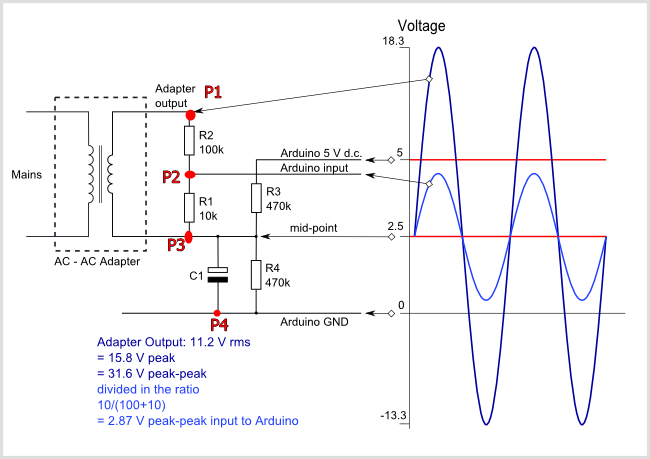Community
OpenEnergyMonitor

# Voltage RMS measurement with Arduino

Is there anyone who can help ?

I try to measure the voltage RMS according to the attached circuit and skecth.
the main questions is why i see 110 Vrms instead of 1,03 Vrms.
Actually i need to know Vrms at the output of AC-AC adaptare

Thanks a lot for helping

Welcome, @umutozkans, to the OEM forum.

You should NOT see 1.03 V rms. You should see the voltage at the input side of the AC-AC Adapter. You did not give us the name of your Country when you created your account here, are you in Istanbul, Turkey? If you are, your voltage is 220 V and the software is designed so that this is the voltage that you should see.

The number you need to adjust is “234.6” in `emon1.voltage(2, 234.6, 1.7)`
 We call that number the ‘calibration factor’. [/edit]

Ordinarily, the number is the mains voltage that will give you exactly 1.0 V at the point where you measured 1.03 V. It will depend on the transformer ratio and the values of R1 & R2, and it is easy to calculate if you know the transformer voltage when it is not loaded - “if” because the transformer will be specified at full load. For example, the EU adapter from our Shop is specified as 9 V (at full load), the actual no-load voltage is 11.5 V.

If you need to know the voltage at the output of the a.c. adapter, the rules are the same - the calibration factor you need is the voltage there that gives you 1.0 V at the Arduino input. In that case, the maths is easy - it is 11.0 ( = (100 kΩ + 10 kΩ) ÷ 10 kΩ).

Finally “Why is it 110 V rms?” – I do not know - it should be close to 220 V, if the voltages you measured are accurate. (But if you read 10.0 V at the adapter output, then your “1.03 V” should be 0.909 V - if the resistor values are correct.)

Exactly right , i want to know adapter output according to input
my some values are like
Main voltage : 220 Vac
R1: 9.8 k R2: 95 k ohm

In this case, I still don’t know how to measure the output value of adapter

Yes you do. Read again what I wrote here:

I have tried this with an emonTx. The resistors are 120 kΩ & 10 kΩ, therefore the calibration constant is 13.0. Setting that in the sketch, I get 11.34 V reported and 11.35 V measured.

Hi Robert, thanks a lot for your interesting but when i change the calibration factor that calculated (9.8+95 )/ 9.8 = 10.69 Nothing has changed. As you can see in the picture, the result is the same again.

I 'm sorry but i couldn’t understand you clearly what you mean.

Have you compiled and uploaded the sketch?

Which sketch is it - screenshots of only part of it don’t help me very much, can you attach the sketch that you are using?

``````#include "EmonLib.h"             // Include Emon Library
EnergyMonitor emon1;             // Create an instance

void setup()
{
Serial.begin(9600);

emon1.voltage(2, 10.69, 1.7);  // Voltage: input pin, calibration, phase_shift
emon1.current(1, 111.1);       // Current: input pin, calibration.
}

void loop()
{
emon1.calcVI(20,2000);         // Calculate all. No.of half wavelengths (crossings), time-out
emon1.serialprint();           // Print out all variables (realpower, apparent power, Vrms, Irms, power factor)

float realPower       = emon1.realPower;        //extract Real Power into variable
float apparentPower   = emon1.apparentPower;    //extract Apparent Power into variable
float powerFActor     = emon1.powerFactor;      //extract Power Factor into Variable
float supplyVoltage   = emon1.Vrms;             //extract Vrms into Variable
float Irms            = emon1.Irms;             //extract Irms into Variable
delay(2000);
}
``````

What you have changed is correct, and it works for me.

I needed to change the pin number for the voltage input because I have loaded the sketch into an emonTx, and the voltage input on that is ‘0’.
I also added another `Serial.print` to check that `supplyVoltage` was filled correctly:

``````#include "EmonLib.h"             // Include Emon Library
EnergyMonitor emon1;             // Create an instance

void setup()
{
Serial.begin(9600);

//  emon1.voltage(2, 10.69, 1.7);  // Voltage: input pin, calibration, phase_shift
//  emon1.current(1, 111.1);       // Current: input pin, calibration.
emon1.voltage(0, 10.69, 1.7);  // Voltage: input pin, calibration, phase_shift
emon1.current(1, 111.1);       // Current: input pin, calibration.

}

void loop()
{
emon1.calcVI(20,2000);         // Calculate all. No.of half wavelengths (crossings), time-out
emon1.serialprint();           // Print out all variables (realpower, apparent power, Vrms, Irms, power factor)

float realPower       = emon1.realPower;        //extract Real Power into variable
float apparentPower   = emon1.apparentPower;    //extract Apparent Power into variable
float powerFActor     = emon1.powerFactor;      //extract Power Factor into Variable
float supplyVoltage   = emon1.Vrms;             //extract Vrms into Variable
float Irms            = emon1.Irms;             //extract Irms into Variable

Serial.print("supplyVoltage = ");  Serial.println(supplyVoltage);  // Added (RW)
delay(2000);
}
``````

and I get (but with no current flowing):

``````0.00 1.56 9.53 0.16 0.00
supplyVoltage = 9.53
-0.00 0.93 9.53 0.10 -0.00
supplyVoltage = 9.53
0.00 0.55 9.53 0.06 0.00
supplyVoltage = 9.53
``````

OK, I have different value resistors, but it is close to the correct value and not 110 V.

Are you sure you have edited, saved and uploaded the correct sketch? I ask this because more than once, I have edited one sketch and uploaded a different sketch, and cannot understand why everything is different and wrong.Do you see any errors when you compile and upload the sketch?

1 Like

some things are getting better.Thanks.
Actually I want to see Vout (P2-P3) not Supply Voltage (P1-P3)

Because of Vout goes to Arduino AI2.
My main purpose is to measure the output voltage…Define what you mean by “output voltage”.
The whole purpose of this circuit is to accurately measure the voltage of the mains electricity supply. If you want to measure something different, then you need to say exactly what it is you want to measure. I cannot guess what is in your head, you must write it down.